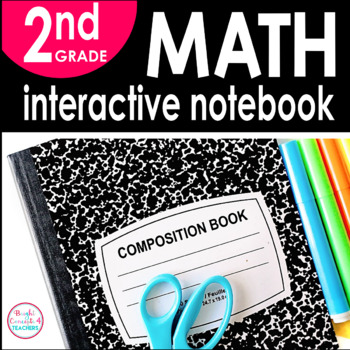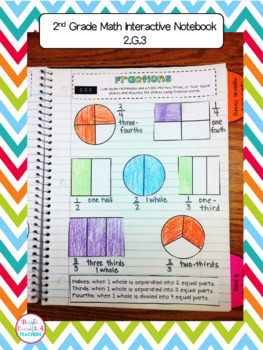# 2nd Grade Math Interactive Notebook {Common Core Aligned}2nd, Homeschool
Subjects
Standards
Resource Type
Formats Included
• PDF
Pages
121 pages

#### Also included in

1. Save with this bundle of SECOND Grade ELA & MATH Interactive Notebooks! This bundle includes the following 3 notebooks from my store:MATH Interactive NotebookLANGUAGE Interactive NotebookREADING Interactive Notebook**If you purchase each notebook separately, it would cost \$38.00. You are getting
\$32.00
\$38.00
Save \$6.00

### Description

Interactive notebooks are a great learning tool in the classroom. They serve as a resource for reviewing standards, a reference tool, or even as an assessment.

This notebook covers all the 2nd Grade Common Core MATH standards (including Operations & Algebraic Thinking, Numbers and Operations in Base 10, Geometry and Measurement and Data)

This product includes:

-Notebook cover

-Divider tabs

-Strand Dividers

-Pictures of EVERY page so you can have an example of how each activity can be used

-Student friendly "I can" statements at the top of each page.

I know your students will love making these notebooks as a method to reinforce all the CCSS math standards they have been taught.

Skills covered:

Operations and Algebraic Thinking:

-Subtraction Strategies

-Problem Solving at various levels

-Doubles and Near Doubles

-Making Ten to Add & Subtract

-Odd and Even

-Arrays

Numbers and Operations in Base 10:

-Place Value

-Skip Counting by 5s, 10s and 100s

-Expanded and Standard Form

-Base 10 Place Value

-Greater than, Less than and Equal

-Problem Solving practice

-Fact Families

-Adding and Subtracting 10 or 100

Geometry:

-Solid Figures

-Plane Shapes

-Rows and Columns

-Equal Parts

-Fractions

-Problem solving practice

Measurement and Data:

-Measuring Length

-Comparing Length

-Number Line

-Problem Solving practice

-Time to 5 minutes

-Money

-Bar Graphs

-Line Plots

-Pictographs

2nd Grade MATH Interactive Notebook BUNDLE

2nd Grade Interactive Notebook BUNDLE {ELA & MATH}

2nd Grade Interactive ELA Notebook BUNDLE

More Math Interactive Notebooks:

KINDERGARTEN Math Interactive Notebook

***************************************************

If you would like to get updates on NEW & CURRENT resources...

**************************************************

Email me at brightconcepts4teachers@gmail.com if you have any questions!

Total Pages
121 pages
N/A
Teaching Duration
1 Year
Report this Resource to TpT
Reported resources will be reviewed by our team. Report this resource to let us know if this resource violates TpT’s content guidelines.

### Standards

to see state-specific standards (only available in the US).
Use addition and subtraction within 100 to solve one- and two-step word problems involving situations of adding to, taking from, putting together, taking apart, and comparing, with unknowns in all positions, e.g., by using drawings and equations with a symbol for the unknown number to represent the problem.
Fluently add and subtract within 20 using mental strategies. By end of Grade 2, know from memory all sums of two one-digit numbers.
Determine whether a group of objects (up to 20) has an odd or even number of members, e.g., by pairing objects or counting them by 2s; write an equation to express an even number as a sum of two equal addends.
Use addition to find the total number of objects arranged in rectangular arrays with up to 5 rows and up to 5 columns; write an equation to express the total as a sum of equal addends.
Recognize and draw shapes having specified attributes, such as a given number of angles or a given number of equal faces. Identify triangles, quadrilaterals, pentagons, hexagons, and cubes.